# Digital Electronics - Combinational Logic Circuits

Exercise : Combinational Logic Circuits - General Questions
6.
Convert BCD 0001 0010 0110 to binary.
1111110
1111101
1111000
1111111
Explanation:
No answer description is available. Let's discuss.

7.
A 74HC147 priority encoder has ten active-LOW inputs and four active-LOW outputs. What would be the state of the four outputs if inputs 4 and 5 are LOW and all other inputs are HIGH?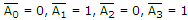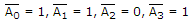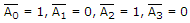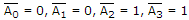Explanation:
No answer description is available. Let's discuss.

8.
Convert BCD 0001 0111 to binary.
10101
10010
10001
11000
Explanation:
No answer description is available. Let's discuss.

9.

Which of the figures in figure (a to d) is equivalent to figure (e)?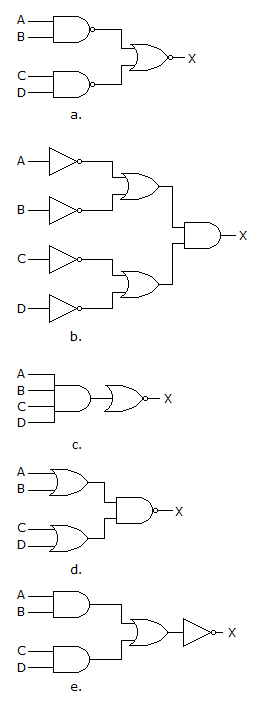a
b
c
d